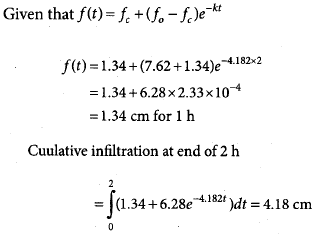Courses

# Test: Hydrology & Irrigation- 1

## 15 Questions MCQ Test GATE Civil Engineering (CE) 2022 Mock Test Series | Test: Hydrology & Irrigation- 1

Description
This mock test of Test: Hydrology & Irrigation- 1 for GATE helps you for every GATE entrance exam. This contains 15 Multiple Choice Questions for GATE Test: Hydrology & Irrigation- 1 (mcq) to study with solutions a complete question bank. The solved questions answers in this Test: Hydrology & Irrigation- 1 quiz give you a good mix of easy questions and tough questions. GATE students definitely take this Test: Hydrology & Irrigation- 1 exercise for a better result in the exam. You can find other Test: Hydrology & Irrigation- 1 extra questions, long questions & short questions for GATE on EduRev as well by searching above.
QUESTION: 1

### A direct runoff hydrograph due to a storm was found to be triangular in shape with a peak of 150 m3/s time from the start of effective storm to the peak of 24 h and a total time base of 72 h. The duration of the storm in this case was

Solution: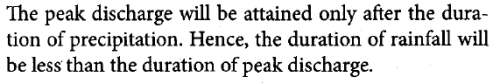QUESTION: 2

### The probability that a 10 year flood will occur at least once in the next 4 years is

Solution: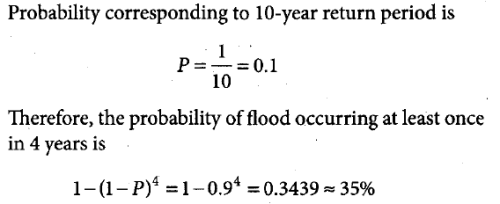QUESTION: 3

### A bridge has an expected life of 50 years and is designed for a flood magnitude of return period 100 years. What of the risk associate with hydrologic design?

Solution: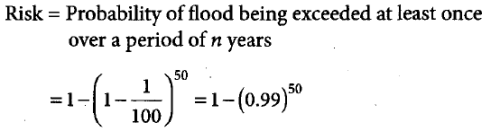QUESTION: 4

A catchment consists of 30% area with runoff coefficient 0.40 and the remaining 70% area with runoff coefficient 0.60. The equivalent runoff coefficient will be

Solution: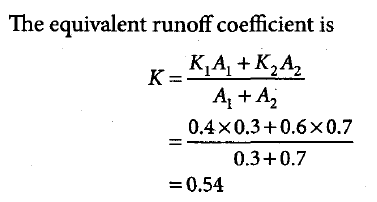*Answer can only contain numeric values
QUESTION: 5

The coefficient of variation of the rainfall for six rain gauge stations in catchments was found to be 29.54%. The optimum number of stations in the catchments for an admissible 10% error in the estimation of the mean rainfall will be

Solution:

The number of optimum stations can be given as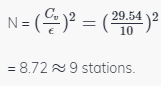QUESTION: 6

If a 4-h hydrograph of a certain basin has a peak ordinate of 80 m3/s, the peak ordinate of a 2-h unit hydrograph for the same basin will be

Solution: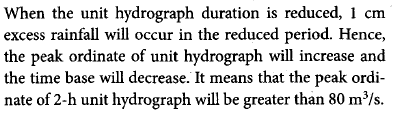QUESTION: 7

A 3 h storm on a small drainage basin produced rainfall intensities of 3.5 cm/h, 4.2 cm/h, and 2.9 cm/h in successive hours. If the surface runoff due to the storm is 3 cm, then the value of Ø -index will be

Solution: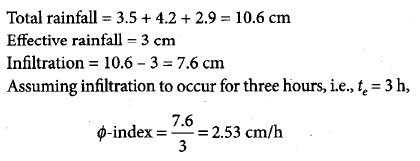QUESTION: 8

A storm with 14 cm precipitation produced a direct runoff of 8 cm. The time distribution of the storm is as shown in the table below: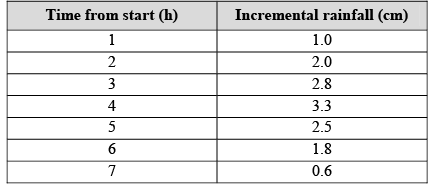What is the value of Ø -index of the storm?

Solution: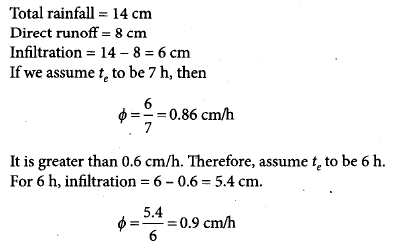*Answer can only contain numeric values
QUESTION: 9

In a river carrying a discharge of 142 m3/s, the stage at station A was 3.6 m and the water surface slope was 1 in 6000. If during a flood, the stage at A was 3.6 m and the water surface slope was 1 in 3000, The flood discharge (approximately) was ________ m3/s.

Solution: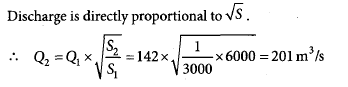QUESTION: 10

A standard, ground-based evaporation pan, corresponding to Indian standards, is installed at the banks of a surface reservoir. The water surface area on a particular day is 100 ha. The recorded evaporation loss from the pan on a certain day is nearly 4 cm. What is the anticipated evaporation loss from the reservoir for the day?

Solution: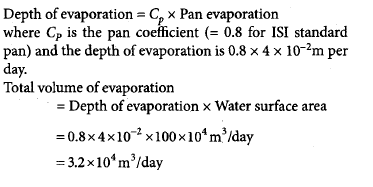QUESTION: 11

The total observed runoff volume during a 4-h storm with a uniform intensity of 2.8 cm/h is 25.2 × 106 m3 from a basin of area 280 km2. What is the average infiltration rate for the basin?

Solution: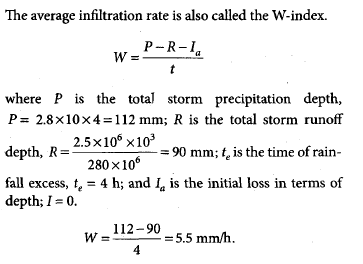QUESTION: 12

A 3-h unit hydrograph U1 of a catchment of area 235 km2 is in the form of triangle with peak discharge of 30 m3/s. Another 3-h unit hydrograph U2 is also triangular in shape and has the same base width as U1, but has a peak flow of 90 m3/s. What is the catchment area of U2?

Solution: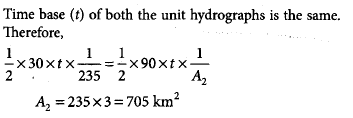*Answer can only contain numeric values
QUESTION: 13

A triangular DRH due to a storm has a time base of 80 h and a peak flow of 50 m3/s occurring at 20 h from the start. If the catchment area is 144 km2, then the rainfall excess in the storm is ________ cm.

Solution:

(Area under DRH) = (Rainfall Excess) x (Catchment Area)

Area under DRH = Area of triangle = 0.5 x Peak Flow (in m3/s) x Time Base (in seconds)
= 0.5 x 50 x 80 x 60 x 60 = 72 x 105 m3
Catchment Area = 144 km2 = 144 x 106 m2
(Area under DRH) = (Rainfall Excess) x (Catchment Area)
Therefore, Rainfall excess = (72 x 105)/ (144 x 106) = 0.05 m = 5cm

QUESTION: 14

The numbers of revolutions of a current meter in 50s were found to be 12 and 30 corresponding to the velocities of 0.25 m/s and 0.46 m/s, respectively. What velocity (in m/s) would be indicated by 50 revolutions of that current meter in 1 min?

Solution: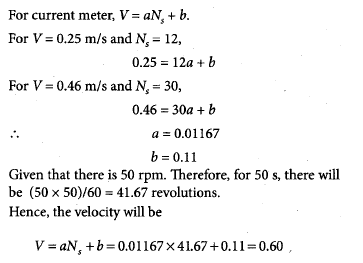QUESTION: 15

The parameters in Horton’s infilteration equation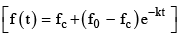are given as f 0 = 7.62 cm/h, f c = 1.34 cm/h, and k = 4.182 h. For assumed continuous ponding, the cumulative infiltration at the end of 2 h is

Solution: